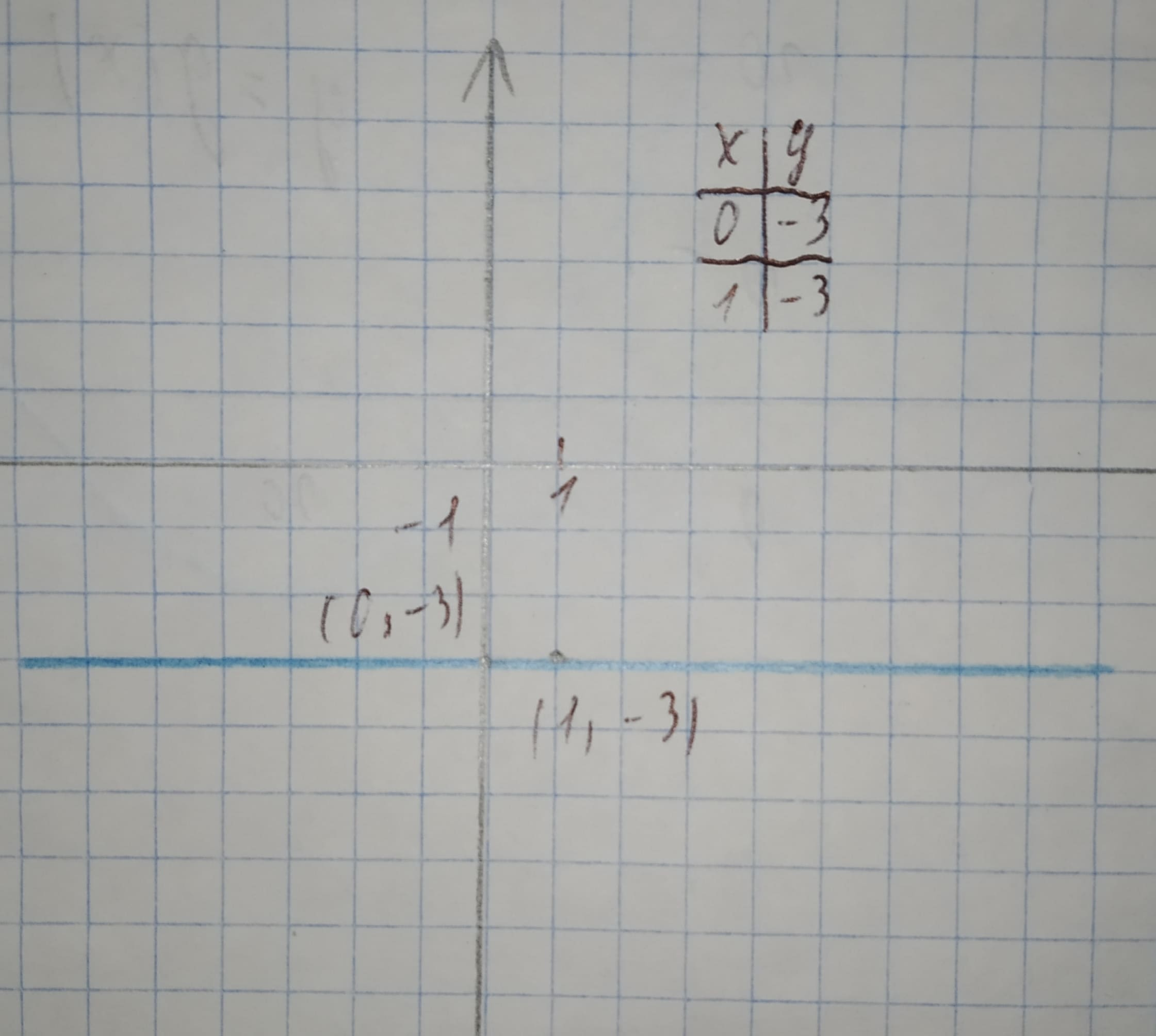Question# We give linear equations. For each equation, a. find the y-intercept and slope. b. determine whether the line slopes upward, slopes downward, or is horizontal, without graphing the equation. c. use two points to graph the equation. y=-3

Linear equations and graphs
ANSWEREDWe give linear equations. For each equation,
a. find the y-intercept and slope.
b. determine whether the line slopes upward, slopes downward, or is horizontal, without graphing the equation.
c. use two points to graph the equation.
$$y=-3$$2021-02-13

a. The y-intercept is the constant in the linear equation:
-3
The slope is the coefficient of x in the linear equation:
0
b. If the slope is positive, then the graph slopes upwards. If the slope is negative, then the graph slopes downwards. If the slope is 0, then the graph is horizontal.
Slope = 0 $$\displaystyle\Rightarrow$$ Horizontal
c. Evaluate the given equation for two values of x.
Graph the two points.
Draw a line through the two points:Results:
a.-3,0
b.Horizontal
c.See graph